Скачать презентацию Simple Interest Warm Up Problem of the Day

6a8e3a6b7168811018700cbd2369d493.ppt

• Количество слайдов: 27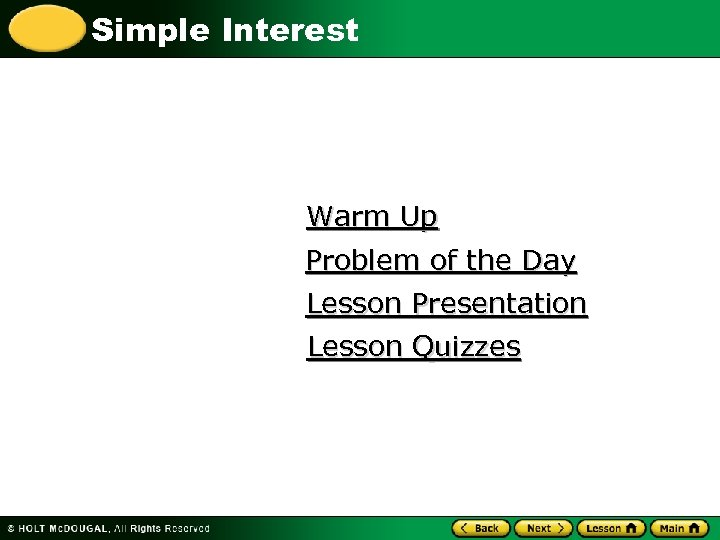Simple Interest Warm Up Problem of the Day Lesson Presentation Lesson Quizzes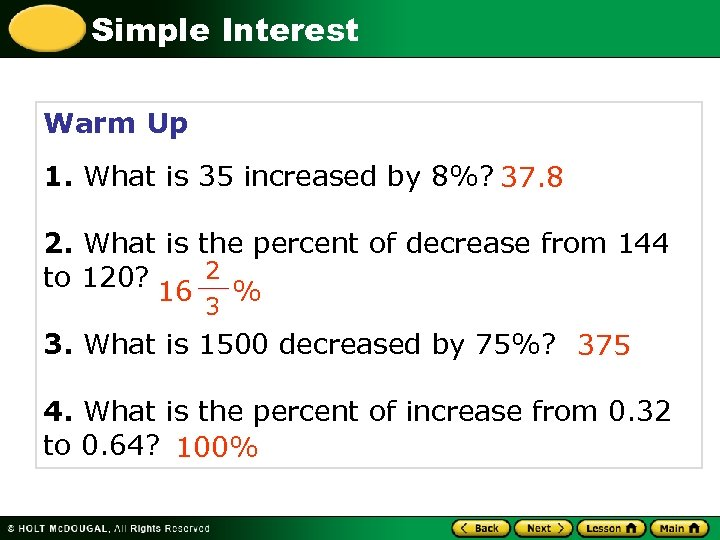Simple Interest Warm Up 1. What is 35 increased by 8%? 37. 8 2. What is the percent of decrease from 144 2 to 120? 16 3 % 3. What is 1500 decreased by 75%? 375 4. What is the percent of increase from 0. 32 to 0. 64? 100%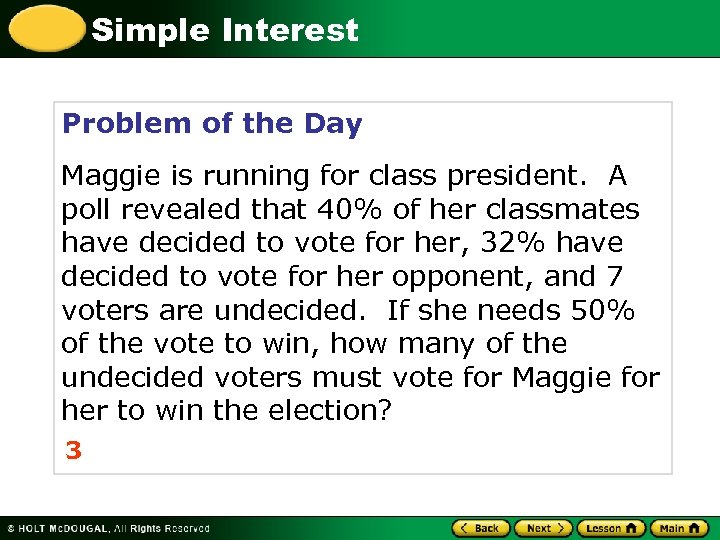Simple Interest Problem of the Day Maggie is running for class president. A poll revealed that 40% of her classmates have decided to vote for her, 32% have decided to vote for her opponent, and 7 voters are undecided. If she needs 50% of the vote to win, how many of the undecided voters must vote for Maggie for her to win the election? 3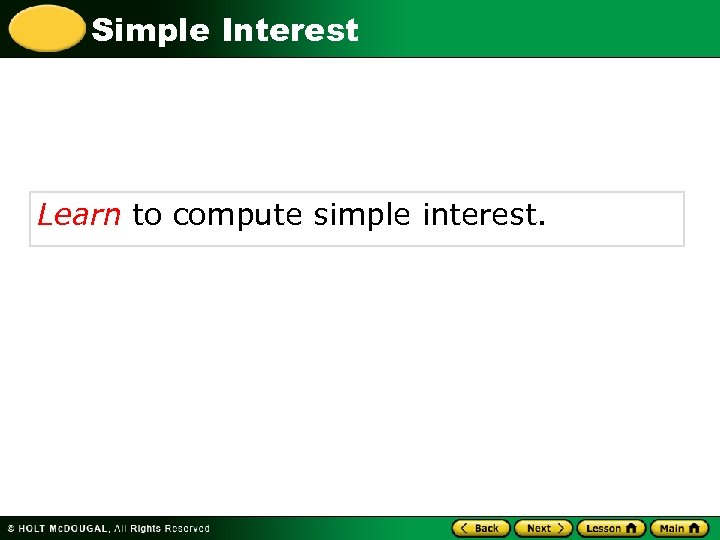Simple Interest Learn to compute simple interest.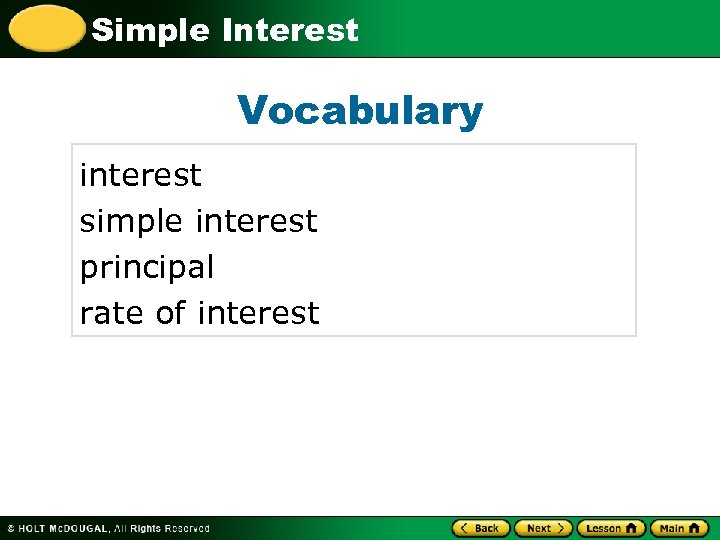Simple Interest Vocabulary interest simple interest principal rate of interest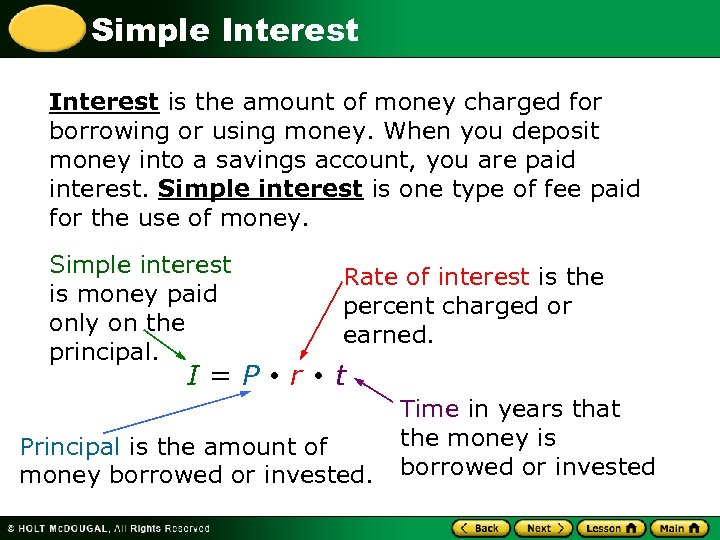Simple Interest is the amount of money charged for borrowing or using money. When you deposit money into a savings account, you are paid interest. Simple interest is one type of fee paid for the use of money. Simple interest is money paid only on the principal. I=P Rate of interest is the percent charged or earned. r t Principal is the amount of money borrowed or invested. Time in years that the money is borrowed or invested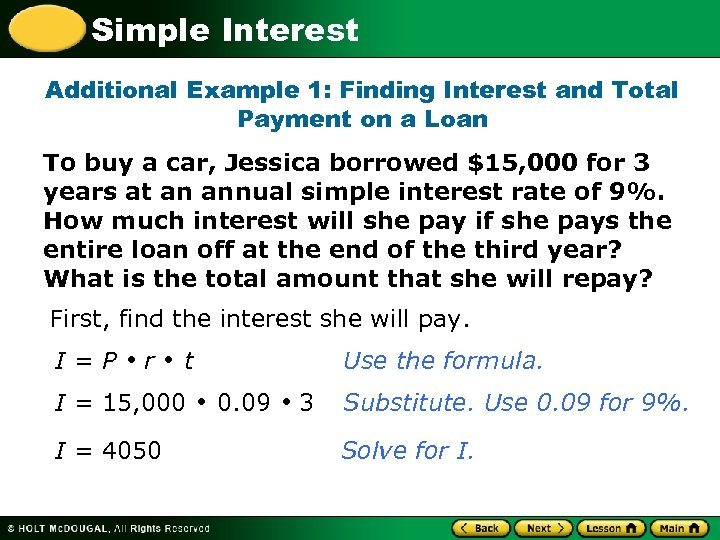Simple Interest Additional Example 1: Finding Interest and Total Payment on a Loan To buy a car, Jessica borrowed \$15, 000 for 3 years at an annual simple interest rate of 9%. How much interest will she pay if she pays the entire loan off at the end of the third year? What is the total amount that she will repay? First, find the interest she will pay. I=P r t I = 15, 000 I = 4050 Use the formula. 0. 09 3 Substitute. Use 0. 09 for 9%. Solve for I.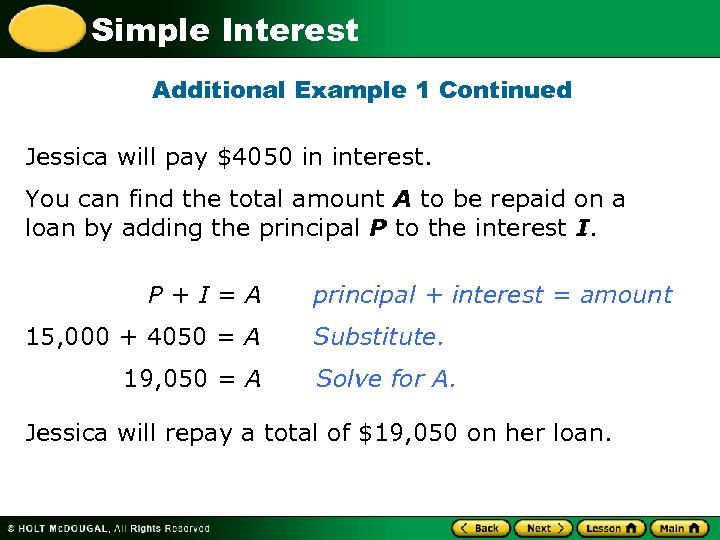Simple Interest Additional Example 1 Continued Jessica will pay \$4050 in interest. You can find the total amount A to be repaid on a loan by adding the principal P to the interest I. P+I=A 15, 000 + 4050 = A 19, 050 = A principal + interest = amount Substitute. Solve for A. Jessica will repay a total of \$19, 050 on her loan.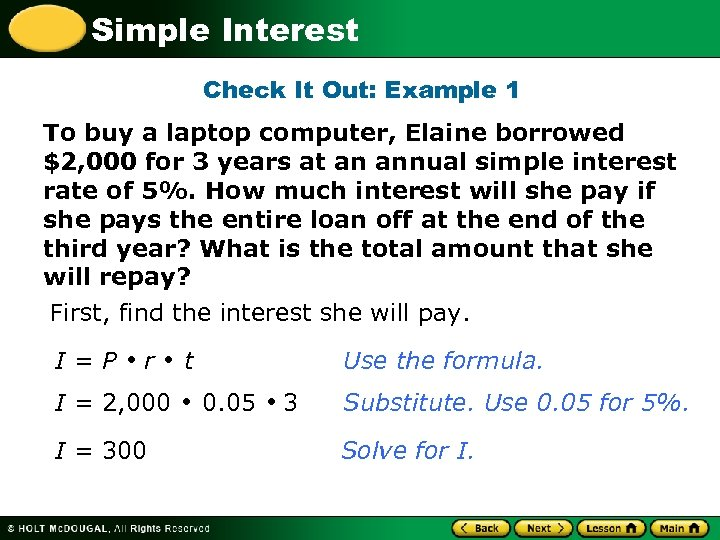Simple Interest Check It Out: Example 1 To buy a laptop computer, Elaine borrowed \$2, 000 for 3 years at an annual simple interest rate of 5%. How much interest will she pay if she pays the entire loan off at the end of the third year? What is the total amount that she will repay? First, find the interest she will pay. I=P r I = 2, 000 I = 300 t Use the formula. 0. 05 3 Substitute. Use 0. 05 for 5%. Solve for I.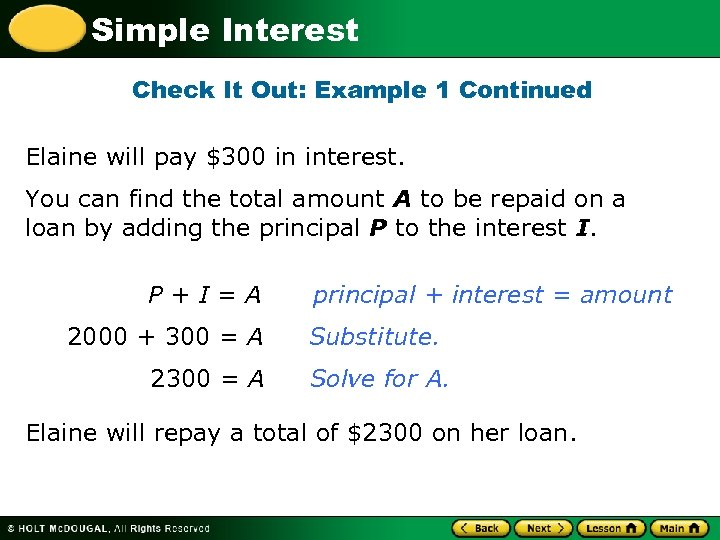Simple Interest Check It Out: Example 1 Continued Elaine will pay \$300 in interest. You can find the total amount A to be repaid on a loan by adding the principal P to the interest I. P+I=A 2000 + 300 = A 2300 = A principal + interest = amount Substitute. Solve for A. Elaine will repay a total of \$2300 on her loan.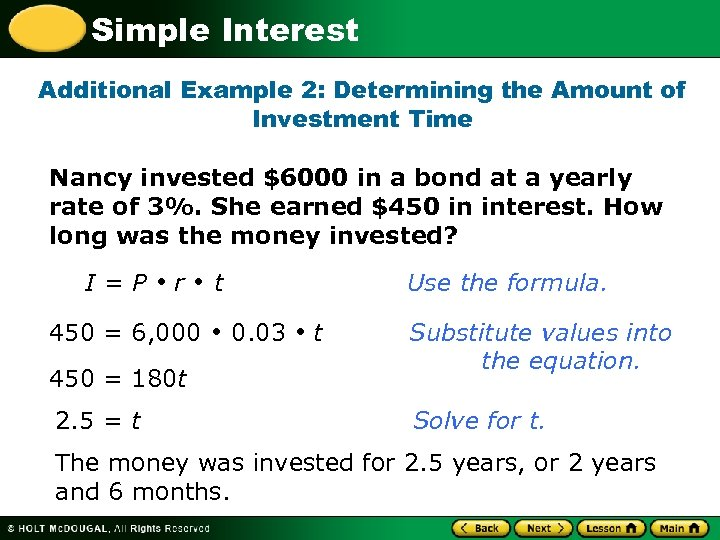Simple Interest Additional Example 2: Determining the Amount of Investment Time Nancy invested \$6000 in a bond at a yearly rate of 3%. She earned \$450 in interest. How long was the money invested? I=P r 450 = 6, 000 450 = 180 t 2. 5 = t t Use the formula. 0. 03 t Substitute values into the equation. Solve for t. The money was invested for 2. 5 years, or 2 years and 6 months.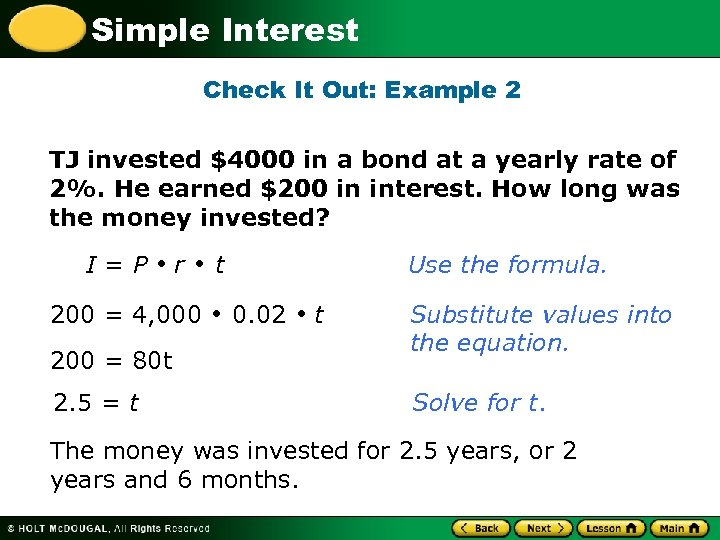Simple Interest Check It Out: Example 2 TJ invested \$4000 in a bond at a yearly rate of 2%. He earned \$200 in interest. How long was the money invested? I=P r 200 = 4, 000 200 = 80 t 2. 5 = t t Use the formula. 0. 02 t Substitute values into the equation. Solve for t. The money was invested for 2. 5 years, or 2 years and 6 months.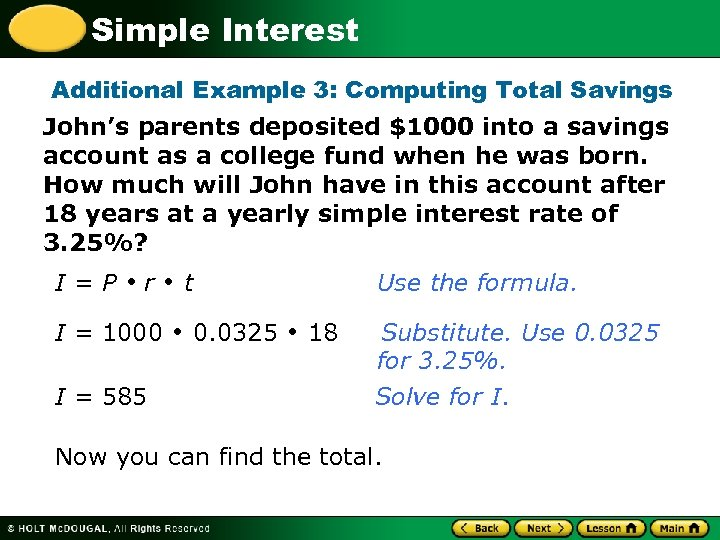Simple Interest Additional Example 3: Computing Total Savings John’s parents deposited \$1000 into a savings account as a college fund when he was born. How much will John have in this account after 18 years at a yearly simple interest rate of 3. 25%? I=P r I = 1000 I = 585 t 0. 0325 Use the formula. 18 Substitute. Use 0. 0325 for 3. 25%. Solve for I. Now you can find the total.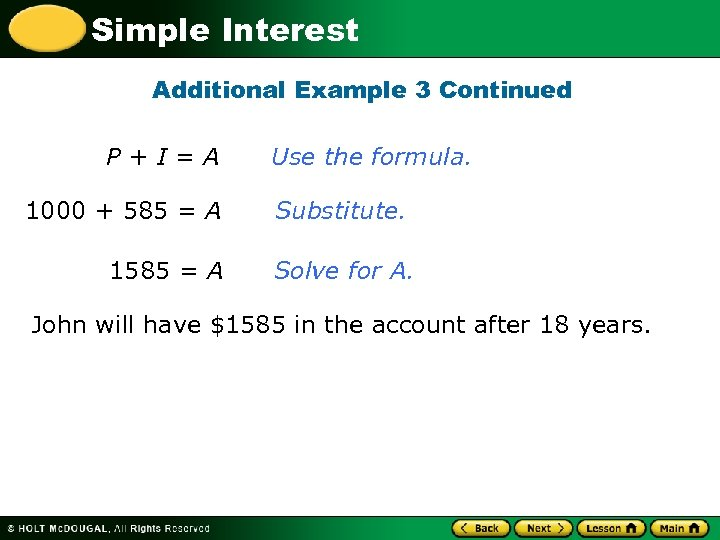Simple Interest Additional Example 3 Continued P+I=A 1000 + 585 = A 1585 = A Use the formula. Substitute. Solve for A. John will have \$1585 in the account after 18 years.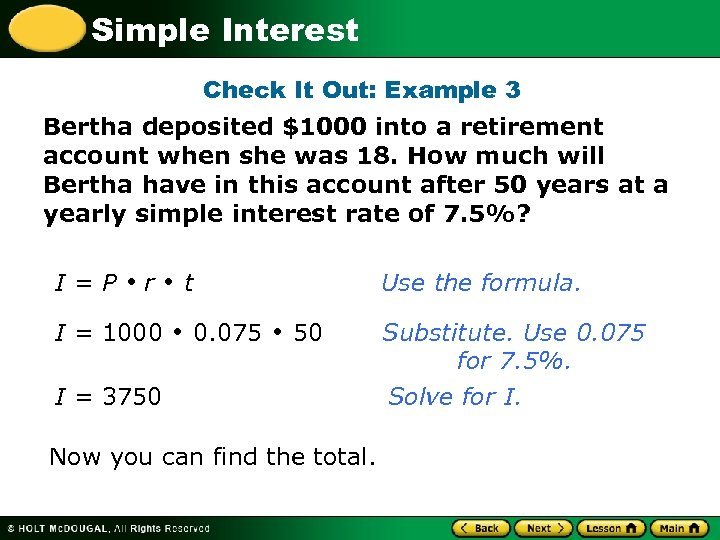Simple Interest Check It Out: Example 3 Bertha deposited \$1000 into a retirement account when she was 18. How much will Bertha have in this account after 50 years at a yearly simple interest rate of 7. 5%? I=P r I = 1000 t 0. 075 Use the formula. 50 I = 3750 Now you can find the total. Substitute. Use 0. 075 for 7. 5%. Solve for I.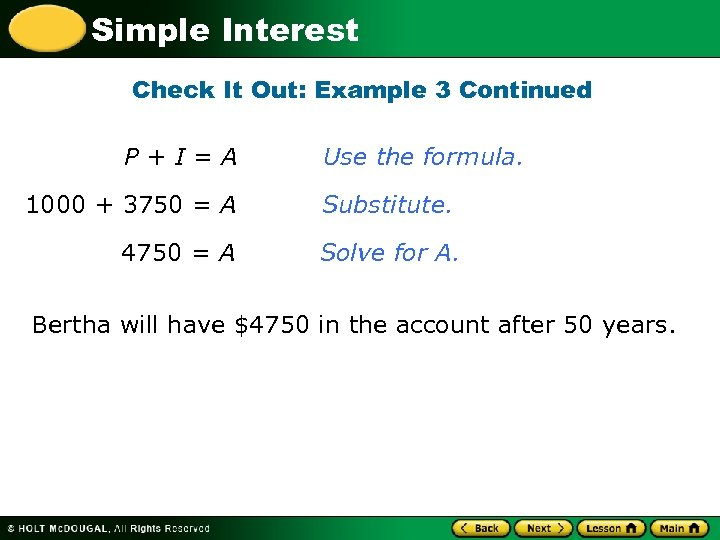Simple Interest Check It Out: Example 3 Continued P+I=A Use the formula. 1000 + 3750 = A Substitute. 4750 = A Solve for A. Bertha will have \$4750 in the account after 50 years.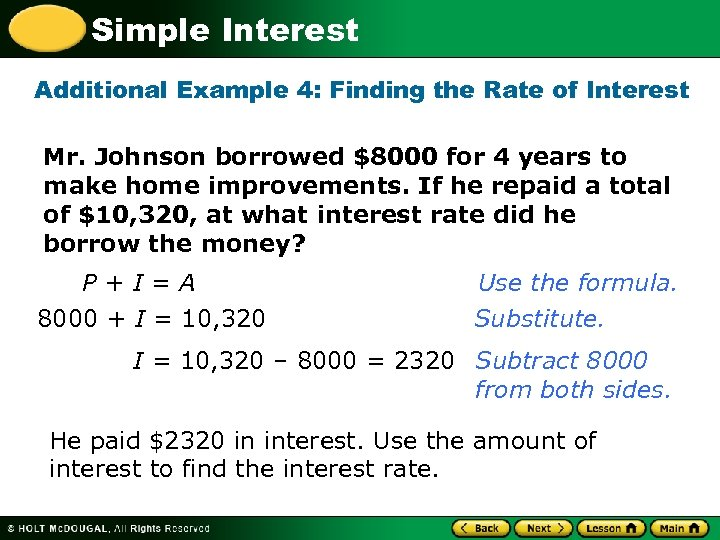Simple Interest Additional Example 4: Finding the Rate of Interest Mr. Johnson borrowed \$8000 for 4 years to make home improvements. If he repaid a total of \$10, 320, at what interest rate did he borrow the money? P+I=A 8000 + I = 10, 320 Use the formula. Substitute. I = 10, 320 – 8000 = 2320 Subtract 8000 from both sides. He paid \$2320 in interest. Use the amount of interest to find the interest rate.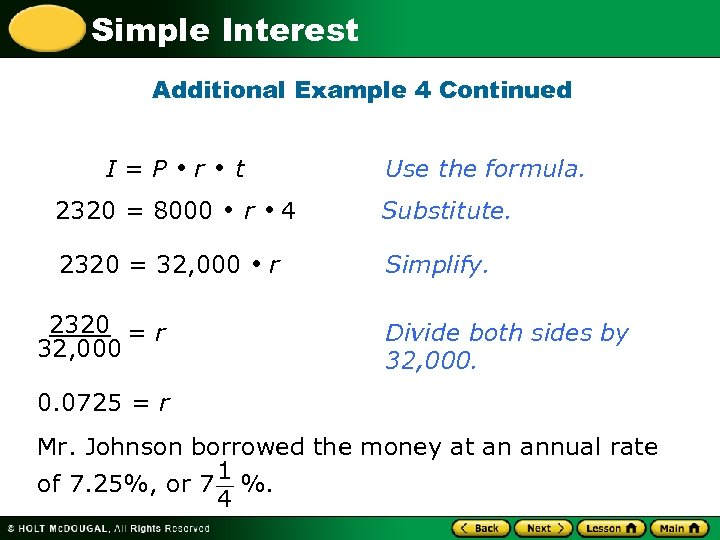Simple Interest Additional Example 4 Continued I=P r 2320 = 8000 t 2320 = 32, 000 2320 = r 32, 000 Use the formula. r r 4 Substitute. Simplify. Divide both sides by 32, 000. 0. 0725 = r Mr. Johnson borrowed the money at an annual rate 1 of 7. 25%, or 7 %. 4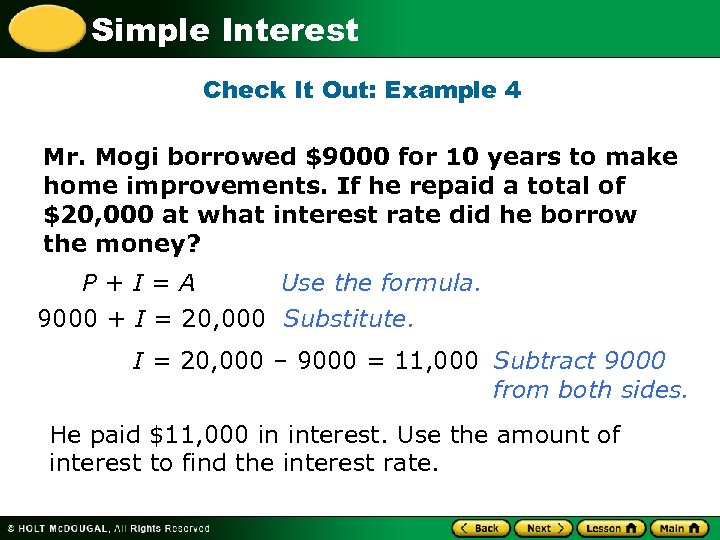Simple Interest Check It Out: Example 4 Mr. Mogi borrowed \$9000 for 10 years to make home improvements. If he repaid a total of \$20, 000 at what interest rate did he borrow the money? P+I=A Use the formula. 9000 + I = 20, 000 Substitute. I = 20, 000 – 9000 = 11, 000 Subtract 9000 from both sides. He paid \$11, 000 in interest. Use the amount of interest to find the interest rate.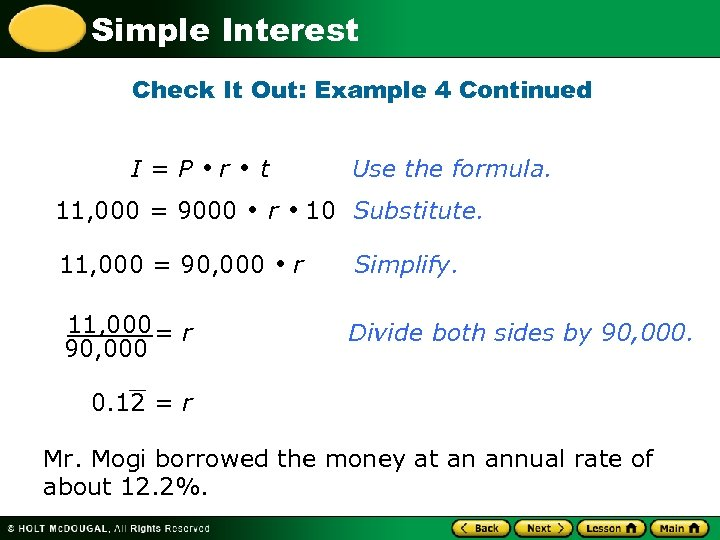Simple Interest Check It Out: Example 4 Continued I=P r 11, 000 = 9000 t 11, 000 = 90, 000 11, 000 = r 90, 000 Use the formula. r r 10 Substitute. Simplify. Divide both sides by 90, 000. 0. 12 = r Mr. Mogi borrowed the money at an annual rate of about 12. 2%.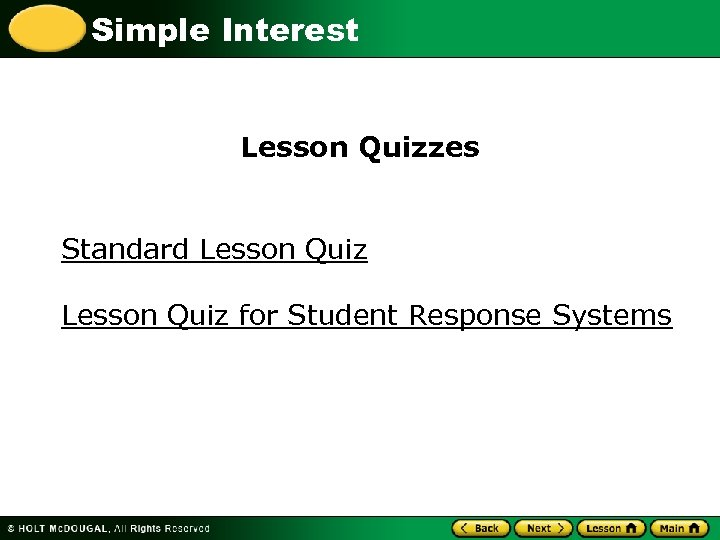Simple Interest Lesson Quizzes Standard Lesson Quiz for Student Response Systems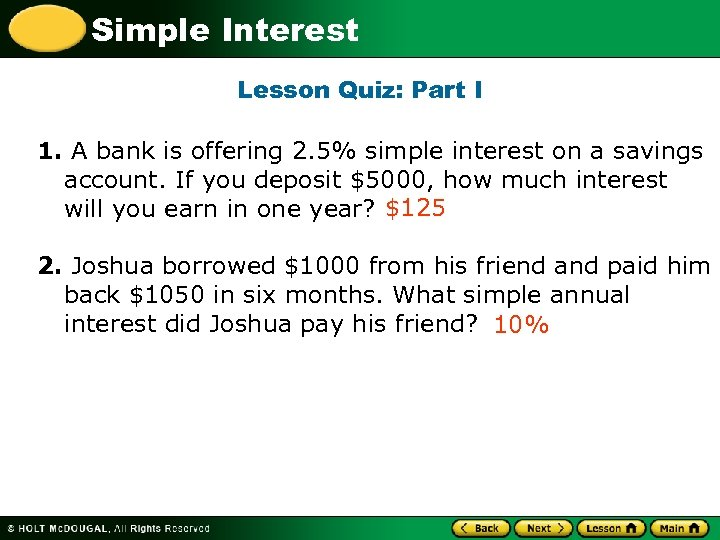Simple Interest Lesson Quiz: Part I 1. A bank is offering 2. 5% simple interest on a savings account. If you deposit \$5000, how much interest will you earn in one year? \$125 2. Joshua borrowed \$1000 from his friend and paid him back \$1050 in six months. What simple annual interest did Joshua pay his friend? 10%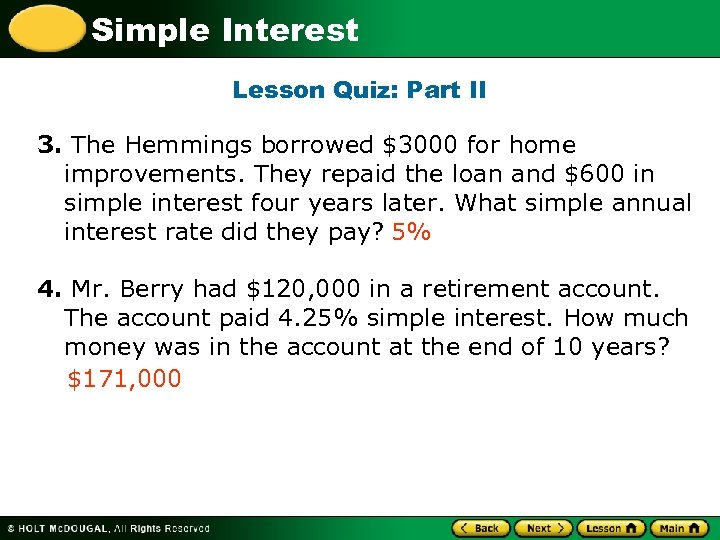Simple Interest Lesson Quiz: Part II 3. The Hemmings borrowed \$3000 for home improvements. They repaid the loan and \$600 in simple interest four years later. What simple annual interest rate did they pay? 5% 4. Mr. Berry had \$120, 000 in a retirement account. The account paid 4. 25% simple interest. How much money was in the account at the end of 10 years? \$171, 000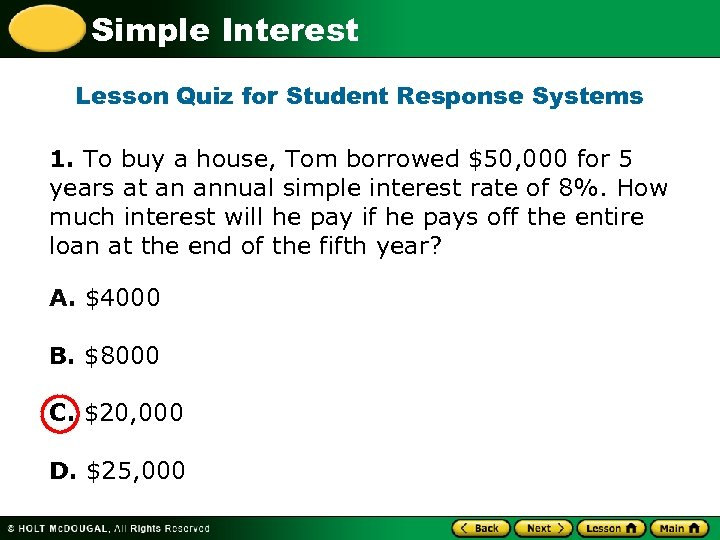Simple Interest Lesson Quiz for Student Response Systems 1. To buy a house, Tom borrowed \$50, 000 for 5 years at an annual simple interest rate of 8%. How much interest will he pay if he pays off the entire loan at the end of the fifth year? A. \$4000 B. \$8000 C. \$20, 000 D. \$25, 000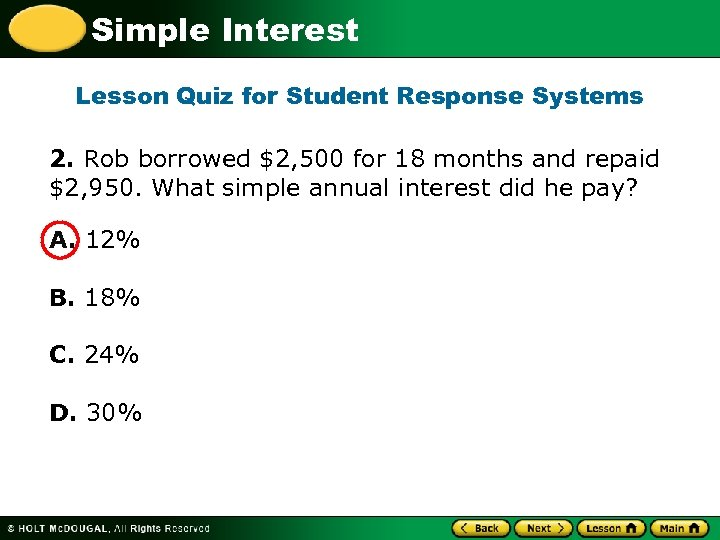Simple Interest Lesson Quiz for Student Response Systems 2. Rob borrowed \$2, 500 for 18 months and repaid \$2, 950. What simple annual interest did he pay? A. 12% B. 18% C. 24% D. 30%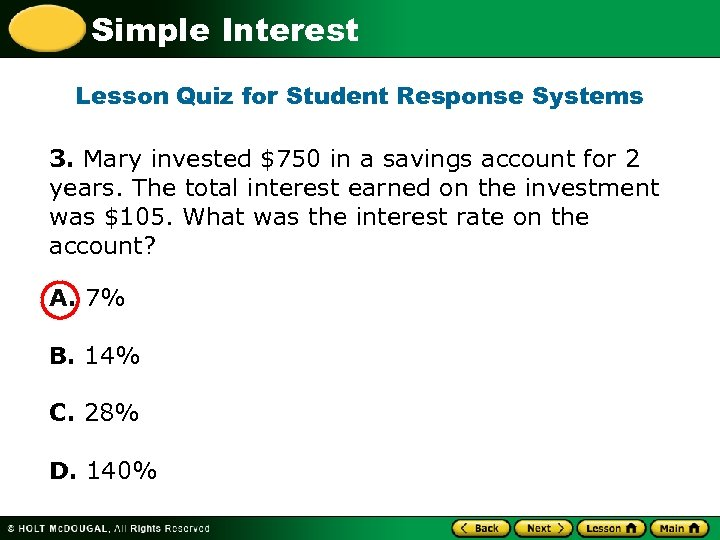Simple Interest Lesson Quiz for Student Response Systems 3. Mary invested \$750 in a savings account for 2 years. The total interest earned on the investment was \$105. What was the interest rate on the account? A. 7% B. 14% C. 28% D. 140%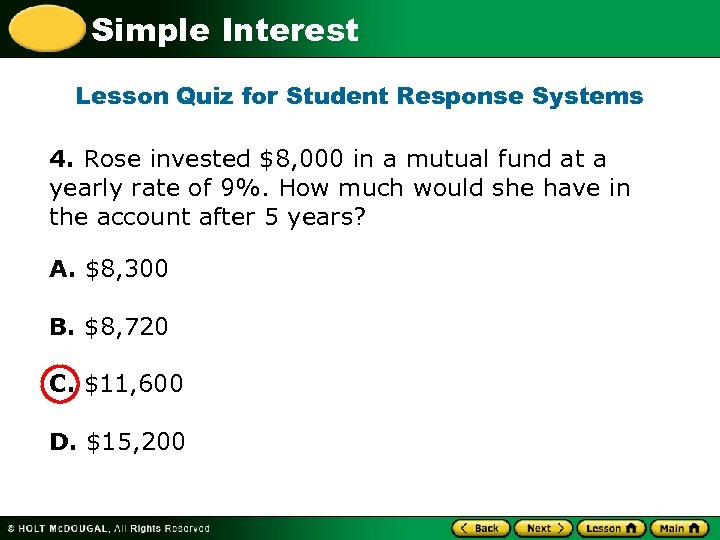Simple Interest Lesson Quiz for Student Response Systems 4. Rose invested \$8, 000 in a mutual fund at a yearly rate of 9%. How much would she have in the account after 5 years? A. \$8, 300 B. \$8, 720 C. \$11, 600 D. \$15, 200